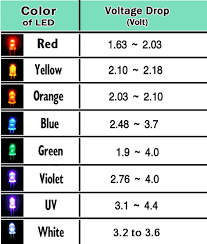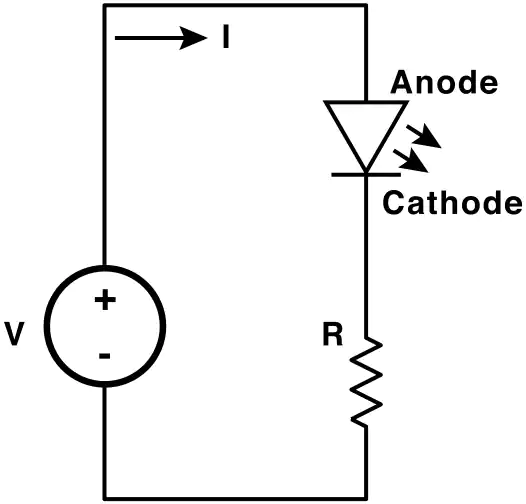Below is some general information for LED usage and voltage drop calculations.

The data sheet that came with your LEDs will tell you the operating voltage and current draw. If this information is not available you can use the table posted here for a general idea of the required voltage.

When calculating the resistor value and wattage ALWAYS go for the nearest higher value if an exact component is not available.

It is possible to adjust the brightness of the LED by changing the resistor value. A lower value will cause the LED to be brighter, and a higher value will cause the LED to be dimmer. This does have it limitations. a value that is to low will cause the LED to burn out. A value that is to high will cause the LED to not turn on.Calculating the needed resistor for the LED and voltage.

Here is the formula for calculating the needed resistor.

R= the needed resistor

Vs = Voltage supplied

Vf = Voltage drop across the LED

If = Forward current used by the LED usually in ma or milliamps

For the following example we have a 12 volt DC power supply. The LED is red and needs 1.8 volts at 20ma.

We will have to calculate for two values. The amount of resistance to adjust the voltage through the LED and the wattage of the resistor to dissipate the heat generated.

Now lets plug in our values. (12-1.8)/.020=510 ohms

12 volts – 1.8 volts used by the LED = 10.2 volts remaining. Now take the 10.2 volts and divide by the current used by the LED which is 20ma which is written .020.   10.2/.020=510 ohms

To calculate the wattage of the resistor use this formula. W=V*I.

W = Wattage

V= Voltage to be dropped

I = Current needed by the LED

We have 10.2 volts to be disposed of and the LED uses 20ma. 10.2 * .020=0.204 watts. To limit the amount of heat generated I always move to the next higher wattage rating. I would use a 1/4 watt resistor for this application.

Correct resistor placement

The resistor should be placed between the Cathode and the negative lead on the power supply.

How to determine the anode and cathode on a 3mm or 5mm through hole LED. If one lead is longer than the other the long lead is the anode or positive lead. If both leads are the same length and the plastic case has a flat spot on the side near one of the leads this is the cathode or negative.